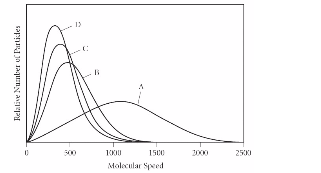# Problem: Using the graph below, determine the gas that has the lowest density at STP.A) AB) BC) CD) DE) All of the gases have the same density at STP.

###### FREE Expert Solution
88% (481 ratings)
###### Problem Details

Using the graph below, determine the gas that has the lowest density at STP.

A) A

B) B

C) C

D) D

E) All of the gases have the same density at STP.What scientific concept do you need to know in order to solve this problem?

Our tutors have indicated that to solve this problem you will need to apply the Standard Temperature and Pressure concept. You can view video lessons to learn Standard Temperature and Pressure. Or if you need more Standard Temperature and Pressure practice, you can also practice Standard Temperature and Pressure practice problems.

What is the difficulty of this problem?

Our tutors rated the difficulty ofUsing the graph below, determine the gas that has the lowest...as medium difficulty.

How long does this problem take to solve?

Our expert Chemistry tutor, Sabrina took 2 minutes and 47 seconds to solve this problem. You can follow their steps in the video explanation above.

What professor is this problem relevant for?

Based on our data, we think this problem is relevant for Professor Donaldson's class at TORONTO.